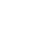18+

18+

18+

18+

18+

18+

18+

18+

18+

18+

18+

18+

18+

18+

18+

18+